# Power Electronics - Choppers

A chopper uses high speed to connect and disconnect from a source load. A fixed DC voltage is applied intermittently to the source load by continuously triggering the power switch ON/OFF. The period of time for which the power switch stays ON or OFF is referred to as the chopper’s ON and OFF state times, respectively.

Choppers are mostly applied in electric cars, conversion of wind and solar energy, and DC motor regulators.

### Symbol of a Chopper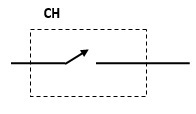### Classification of Choppers

Depending on the voltage output, choppers are classified as −

• Step Up chopper (boost converter)
• Step Down Chopper(Buck converter)
• Step Up/Down Chopper (Buck-boost converter)

## Step Up Chopper

The average voltage output (Vo) in a step up chopper is greater than the voltage input (Vs). The figure below shows a configuration of a step up chopper.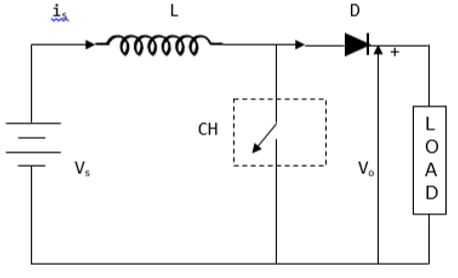### Current and Voltage Waveforms

V0 (average voltage output) is positive when chopper is switched ON and negative when the chopper is OFF as shown in the waveform below.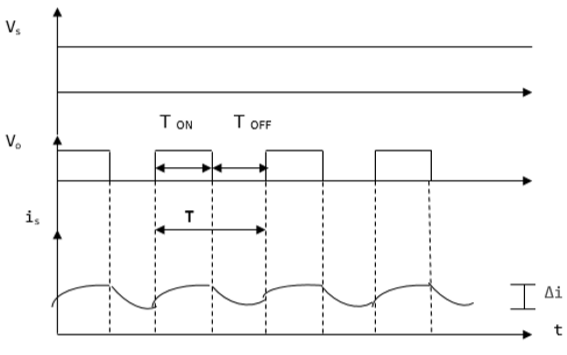Where

TON – time interval when chopper is ON

TOFF – time interval when chopper is OFF

Vs – Source voltage

T – Chopping time period = TON + TOFF

Vo is given by −

$$V_{0}=\frac{1}{T}\int_{0}^{T_{ON}}V_{S}dt$$

When the chopper (CH) is switched ON, the load is short circuited and, therefore, the voltage output for the period TON is zero. In addition, the inductor is charged during this time. This gives VS = VL

$L\frac{di}{dt}=V_{S},$ $\frac{\Delta i}{T_{ON}}=\frac{V_{S}}{L}$

Hence,$\Delta i=\frac{V_{S}}{L}T_{ON}$

Δi = is the inductor peak to peak current. When the chopper (CH) is OFF, discharge occurs through the inductor L. Therefore, the summation of the Vs and VL is given as follows −

$V_{0}=V_{S}+V_{L},\quad V_{L}=V_{0}-V_{S}$

But $L\frac{di}{dt}=V_{0}-V_{S}$

Thus,$L\frac{\Delta i}{T_{OFF}}=V_{0}-V_{S}$

This gives,$\Delta i=\frac{V_{0}-V_{S}}{L}T_{OFF}$

Equating Δi from ON state to Δi from OFF state gives −

$\frac{V_{S}}{L}T_{ON}=\frac{V_{0}-V_{S}}{L}T_{OFF}$, $V_{S}\left ( T_{ON}+T_{OFF} \right )=V_{0}T_{OFF}$

$V_{0}=\frac{TV_{S}}{T_{OFF}}=\frac{V_{S}}{\frac{\left ( T+T_{ON} \right )}{T}}$

This give the average voltage output as,

$$V_{0}=\frac{V_{S}}{1-D}$$

The above equation shows that Vo can be varied from VS to infinity. It proves that the output voltage will always be more than the voltage input and hence, it boosts up or increases the voltage level.

## Step Down Chopper

This is also known as a buck converter. In this chopper, the average voltage output VO is less than the input voltage VS. When the chopper is ON, VO = VS and when the chopper is off, VO = 0

When the chopper is ON −

$V_{S}=\left ( V_{L}+V_{0} \right ),\quad V_{L}=V_{S}-V_{0},\quad L\frac{di}{dt}=V_{S}-V_{0},\quad L\frac{\Delta i}{T_{ON}}=V_{s}+V_{0}$

Thus, peak-to-peak current load is given by,

$\Delta i=\frac{V_{s}-V_{0}}{L}T_{ON}$

### Circuit Diagram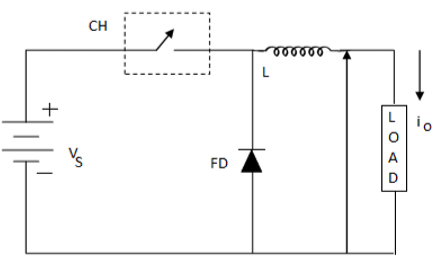Where FD is free-wheel diode.

When the chopper is OFF, polarity reversal and discharging occurs at the inductor. The current passes through the free-wheel diode and the inductor to the load. This gives,

$$L\frac{di}{dt}=V_{0}........................................\left ( i \right )$$

Rewritten as −$\quad L\frac{\Delta i}{T_{OFF}}=V_{0}$

$$\Delta i=V_{0}\frac{T_{OFF}}{L}...................................\left ( ii \right )$$

Equating equations (i) and (ii) gives;

$\frac{V_{S}-V_{0}}{L}T_{ON}=\frac{V_{0}}{L}T_{OFF}$

$\frac{V_{S}-V_{0}}{V_{0}}=\frac{T_{OFF}}{T_{ON}}$

$\frac{V_{S}}{V_{0}}=\frac{T_{ON}-T_{OFF}}{T_{ON}}$

The above equation gives;

$$V_{0}=\frac{T_{ON}}{T}V_{S}=DV_{S}$$

Equation (i) gives −

$\Delta i=\frac{V_{S}-DV_{S}}{L}DT$, from $D=\frac{T_{ON}}{T}$

$=\frac{V_{S}-\left ( 1-D \right )D}{Lf}$

$f=\frac{1}{T}=$chopping frequency

### Current and Voltage Waveforms

The current and voltage waveforms are given below −

For a step down chopper the voltage output is always less than the voltage input. This is shown by the waveform below.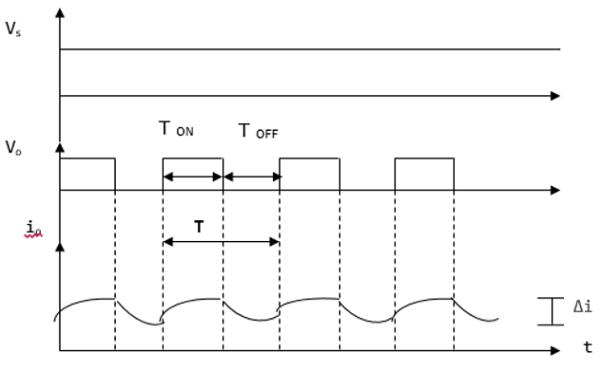## Step Up/ Step Down Chopper

This is also known as a buck-boost converter. It makes it possible to increase or reduce the voltage input level. The diagram below shows a buck-boost chopper.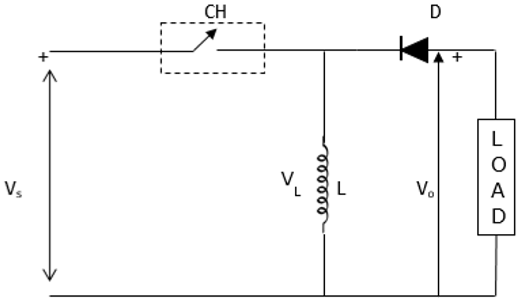When the chopper is switched ON, the inductor L becomes charged by the source voltage Vs. Therefore, Vs = VL.

$$L\frac{di}{dt}=V_{S}$$ $$\Delta i=\frac{V_{S}}{L}T_{ON}=\frac{V_{S}}{L}T\frac{T_{ON}}{T}=\frac{DV_{S}}{Lf}$$

Because −

$D=\frac{T_{ON}}{T}$ and $f=\frac{1}{T} .............................................. \left ( iii \right )$

When the chopper is switched OFF, the inductor’s polarity reverses and this causes it to discharge through the diode and the load.

Hence,

$$V_{0}=-V_{L}$$ $$L\frac{di}{dt}=-V_{0}$$

$L\frac{\Delta i}{T_{OFF}}=-V_{0}$, thus $\Delta i=-\frac{V_{0}}{L}T_{OFF}................................\left ( iv \right )$

Evaluating equation (iii) and (iv) gives −

$\frac{DV_{S}}{Lf}=-\frac{V_{0}}{L}T_{OFF}$, $DV_{S}=-DV_{S}=-V_{0}T_{OFF}f$

$DV_{S}=-V_{0}\frac{T-T_{ON}}{T}=-V_{0}\left ( 1-\frac{T_{ON}}{T} \right )$, $V_{0}=-\frac{DV_{S}}{1-D}$

Because $D=\frac{T_{ON}}{T}=\frac{T-T_{OFF}}{1-D}$

This gives,

$V_{0}=\frac{DV_{S}}{1-D}$

D can be varied from 0 to 1. When, D = 0; VO = 0

When D = 0.5, VO = VS

When, D = 1, VO = ∞.

Hence, in the interval 0 ≤ D ≤ 0.5, output voltage varies in the range 0 ≤ VO < VS and we get step down or Buck operation. Whereas, in the interval 0.5 ≤ D ≤ 1, output voltage varies in the range VS ≤ VO ≤ ∞ and we get step up or Boost operation.